# Di and polybasic acids and bases

We use so many acids and bases every day, such as vinegar or acetic acid in the kitchen, boric acid for laundry, baking soda for the purpose of cooking, washing soda for cleaning etc. Many of the acids, that we do not consume in the household are used in the laboratories, which includes acids such as HCl, H2SO4 etc. and bases such as NaOH, KOH etc. Some of these acids and bases have a single hydronium ion or a hydroxyl ion to shed, but most of them have multiple ions. In this section, we will learn about acids and bases which contain more than one ionizable ion per molecule.

Polybasic acids

Taking the example of a few acids such as sulphuric acid, phosphoric acid, we can see that they contain more than one ionizable ion per molecule. Such acids are termed as polybasic acids.

Let us consider the following ionization reaction of a typical polybasic acid.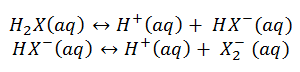This is an example of dissociation of a dibasic acid into its constituent ions.

The equilibrium constant for the above reaction can be given as,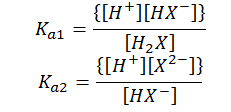Here, Ka1 and Ka2 are the first and the second ionization constants of the acid H2X.

Similarly, for a tribasic acid we have three ionization constants Ka1, Ka2 and Ka3 and so on.

Poly-acidic bases

Taking the example of a few bases such as calcium hydroxide, aluminium hydroxide, we can see that they contain more than one ionizable ion per molecule of base. Such acids are termed as poly-acidic bases.

Let us consider the following ionization reaction of a typical poly-acidic base.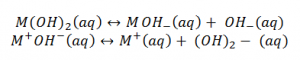, this is an example of dissociation of a diacidic base into its constituent ions.

The equilibrium constant for the above reaction can be given as,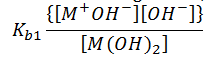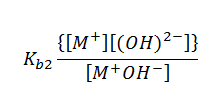Here, Kb1 and Kb2 are the first and the second ionization constants of the base M(OH)2.

Similarly, for a triacidic base we have three ionization constants Ka1, Ka2 and Ka3 and so on.